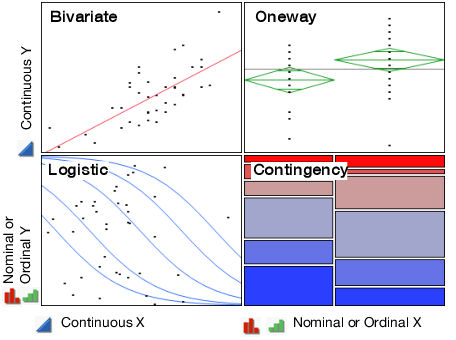Basic Analysis > Introduction to Fit Y by X
Publication date: 07/30/2020

# Introduction to Fit Y by X

##### Examine Relationships between Two Variables

The Fit Y by X platform analyzes the pair of X and Y variables that you specify, by context, based on modeling type.

Here are the four types of analyses:

Bivariate fitting

One-way analysis of variance

Logistic regression

Contingency table analysis

Figure 4.1 Examples of Four Types of Analyses